# Primitive root

(diff) ← Older revision | Latest revision (diff) | Newer revision → (diff)

A primitive root of unity of orderin a fieldis an elementofsuch thatand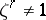for any positive integer. The element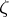generates the cyclic group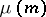of roots of unity of order.

If inthere exists a primitive root of unity of order, thenis relatively prime to the characteristic of. An algebraically closed field contains a primitive root of any order that is relatively prime with its characteristic. Ifis a primitive root of order, then for anythat is relatively prime to, the element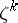is also a primitive root. The number of all primitive roots of orderis equal to the value of the Euler function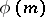if.

In the field of complex numbers, the primitive roots of ordertake the form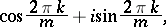where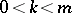andis relatively prime to.

A primitive root modulois an integersuch thatfor, whereis the Euler function. For a primitive root, its powers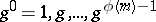are incongruent moduloand form a reduced residue system modulo(cf. Reduced system of residues). Therefore, for each numberthat is relatively prime toone can find an exponentfor which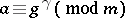.

Primitive roots do not exist for all moduli, but only for moduliof the form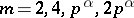, where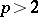is a prime number. In these cases, the multiplicative groups (cf. Multiplicative group) of reduced residue classes modulohave the simplest possible structure: they are cyclic groups of order. The concept of a primitive root modulois closely related to the concept of the index of a number modulo.

Primitive roots modulo a prime number were introduced by L. Euler, but the existence of primitive roots modulo an arbitrary prime number was demonstrated by C.F. Gauss (1801).

How to Cite This Entry:
Primitive root. Encyclopedia of Mathematics. URL: http://encyclopediaofmath.org/index.php?title=Primitive_root&oldid=18612
This article was adapted from an original article by L.V. Kuz'minS.A. Stepanov (originator), which appeared in Encyclopedia of Mathematics - ISBN 1402006098. See original article